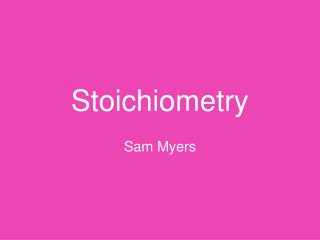DownloadDownload PresentationStoichiometry

# Stoichiometry

Télécharger la présentation## Stoichiometry

- - - - - - - - - - - - - - - - - - - - - - - - - - - E N D - - - - - - - - - - - - - - - - - - - - - - - - - - -
##### Presentation Transcript

1. Stoichiometry Sam Myers

2. How much ammonium hydroxide is needed to react completely with 75.0 g of copper (II) nitrate? NH4OH +Cu(NO3)2 ---> Cu(OH)2 + 2 NH4NO3

3. Stoichiometry First Step: Take the amount you are giving in the question and create an equation. Multiply the given by 1 Mole and divide that by the molar mass of the given. 75.0g x 1 mole / 187.6g

4. Stoichiometry Next you multiply your answer by the ratio of the equation. What you are trying to find over what you know. The answer to 75.0g/ 187.6g was .39 In the equation for Ammonium hydroxide there was a 2 in front of it which means that goes on top of the ration, with the copper (II) chloride there was a 1 so that goes on the bottom. .53 x 2/1

5. Stoichiometry Now you multiply .53 by 2, then divide by 1. The answer is 1.06. Next you multiply that by the molar mass of what were trying to find which is the ammonium hydroxide which is 63.55. The answer is 27.3 g of NH4OH